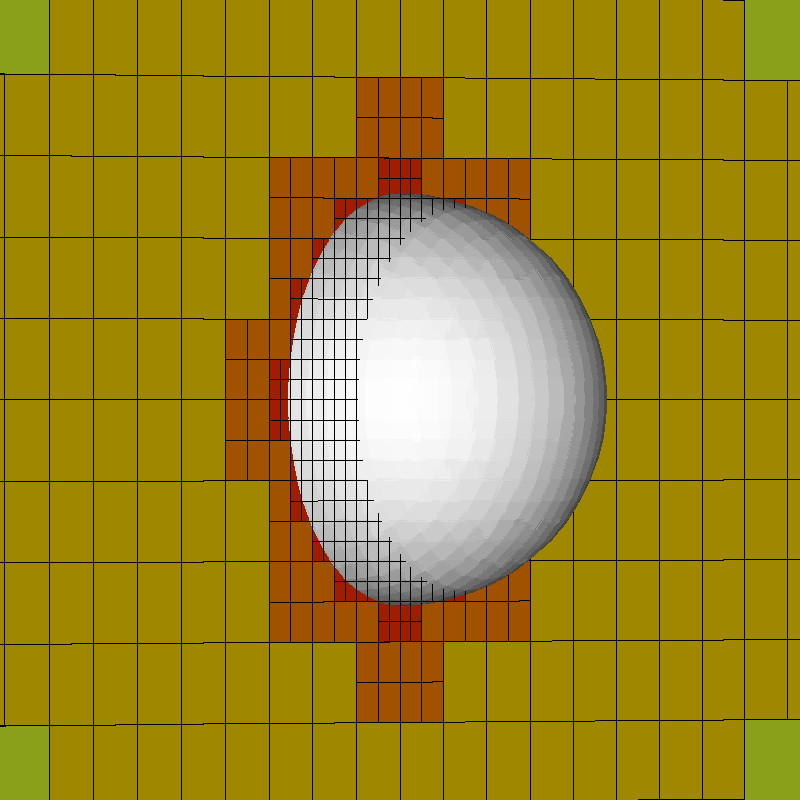# Modification of Basilisk View Test

Note that this is not “really” a bug. The offset is there so that the lines are visible “on top” of squares or other surfaces. This could be improved by making this offset a function of the zoom level.

#include "grid/octree.h"
#include "fractions.h"
#include "view.h"

#define L 100.
#define R 1.

int main() {

We define a volume fraction field much smaller than the domain size.

  size (L);
init_grid (32);
origin (-L/2.,-L/2.,-L/2.);
refine (sq(x) + sq(y) + sq(z) - sq(R*4.) < 0 && level < 8);
refine (sq(x) + sq(y) + sq(z) - sq(R*1.1) < 0 && level < 10);

Refine locally around the sphere.

  scalar f[];
fraction (f, sq(x) + sq(y) + sq(z) - sq(R));

Then display it using Basilisk view functions. Lines from the cells visualization option appear to intersect the VOF interface at a different plane than the Level squares visualization, despite both being at z=0.Misalignment between grid and interface

  view (fov = 0.74, quat = {0,-0.48,0,0.88}, tx = 0, ty = 0, width = 800, height = 800, samples = 1);
box();
draw_vof("f");
squares("level",min=0,max=12);
cells();

save ("out.png");
}